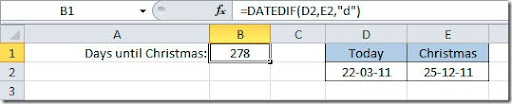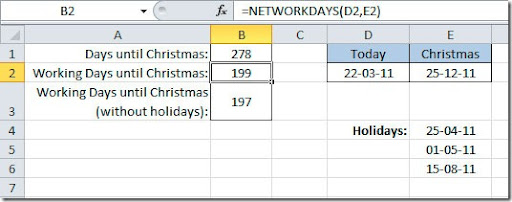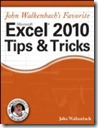### Calculating the difference between two dates

The easiest way to calculate the difference between two dates in Excel is to use the DATEDIF() function. The syntax is like this:

DATEDIF(start_date, end_date,unit)

start_date and end_date are just the starting date and finishing date from where you want to calculate the difference. unit is the date unit to be used on the result. It can be:

y – number of years between start_date and end_date
m
– number of months between start_date and end_date
d
– number of days between start_date and end_date
md
– difference in the day component between start_date and end_date. This means that the years and months are not included in the calculation
ym – difference in the month component between start_date and end_date. This means that the years and days are not included in the calculation
yd – number of days between start_date and end_date, with the year components excluded from the calculation

So, to give you an example of this function works, lets calculate how many days from now until Christmas.We are calculation the difference between today (cell D2) and Christmas day (cell E2). This tell us that there are 278 days until Christmas. We could calculate how many working days are left until Christmas. For that we could use the NETWORKDAYS() function:

NETWORKDAYS(start_date, end_date, holidays)

start_date and end_date are just the starting date and finishing date from where you want to calculate the difference. holidays are the dates to exclude from this because they are not working days, they are public holidays. You can set a range where you put this holidays to be excluded. On this next example we have on cell B2 a formula to calculate just the working days until Christmas that gives us 199 days.On cell B3 we have the formula:

=NETWORKDAYS(D2, E2, E4:E6)

This will also calculate the number of working days between today value on cell D2 and Christmas day on cell E2 but excluding the dates that you put on range E4:E6 that can be public holidays or days where you don’t work for some reason. On this case, as 1 May is a Sunday, it is already excluded from the NETWORKDAYS calculation, the formula will only exclude the other 2 public holidays giving us a result of 197 working days until Christmas.

### Recommended Book - John Walkenbach's Favorite Excel 2010 Tips and TricksThis is a great book from John Walkenbach, also known as “Mr. Spreadsheet”, that will teach you some tricks and will give you great tips on how to do things in Excel 2010. With John Walkenbach's Favorite Excel 2010 Tips and Tricks book you'll get a jump start on mastering the extensive changes to the 2010 version of Excel. This is in my opinion ones of the top books that you should have if you want to master Excel, specially, the 2010 version.